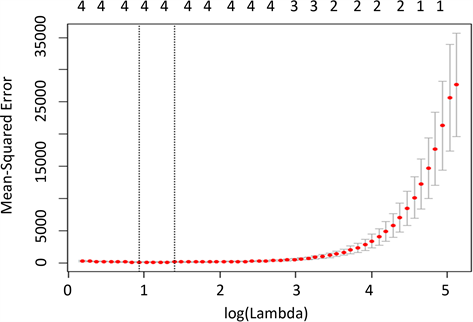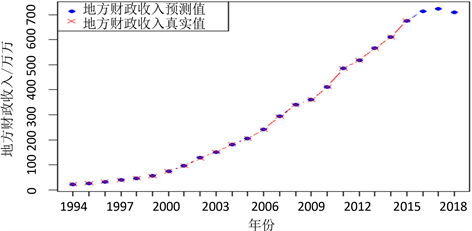﻿ 基于数据挖掘技术的温州市财政收入的影响因素分析及预测

基于数据挖掘技术的温州市财政收入的影响因素分析及预测Analysis and Prediction of Influencing Factors of Fiscal Revenue in Wenzhou Based on Data Mining Technology

Abstract: This paper established a Lasso model, which selects four key factors affecting the financial reve-nue of Wenzhou, such as the total wages of staff and workers, per capita consumption expenditure of urban residents, GDP and tax. On this basis, we also get the forecast values of above four factors especially from 2016 to 2018 by using GM (1, 1) model. Finally, we give the forecast values of financial revenue of Wenzhou from 2016 to 2018 by building an appropriate BP neural network model.

1. 引言

2. 变量选取和数据初探

2.1. 变量选取

${x}_{1}$ ：在岗职工工资总额(亿元)，指一定时期内职工的工资水平，可以用来反映社会分配情况。

${x}_{2}$ ：社会消费品零售总额(亿元)，反映了社会整体消费状况。通常人们的消费需求扩大会引起税收以及经济系统中其他方面发生变化，从而拉动财政收入的增长。

${x}_{3}$ ：城镇居民人均可支配收入(元)，城镇居民人均可支配收入越高就表示人们能用于平常生活中的收入越高，日常消费越大，财政收入的增加值也就越多。

${x}_{4}$ ：城镇居民人均消费性支出(元)，城镇居民人均消费支出越大说明人们的消费水平越高，消费的越多，财政收入也会相应增加。

${x}_{5}$ ：年末总人口(万人)，人口和经济的关系是双向的，在其余因素不变的情况下，地方总人口数越多，人均财政收入越少。

${x}_{6}$ ：全社会固定资产投资额(亿元)，是社会固定资产再生产的主要手段，能调整经济结构，增强经济实力，在一定程度上影响着财政收入。

${x}_{7}$ ：地区生产总值(亿元)，等于各行业增加值的总和，反映一个地区的经济发展水平，通常地区经Table 1. Financial revenue and related data of Wenzhou

${x}_{8}$ ：第一产业总值(亿元)，随着我国产业结构的转型升级，第一产业总值占生产总值的比例减小，对财政收入的影响日益减弱。

${x}_{9}$ ：税收(亿元)，税收是财政收入的主要来源。

${x}_{10}$ ：第三产业与第二产业产值比，表示温州市的现有产业结构，产业结构的优化会刺激财政收入的增长。

2.2. 数据描述性分析

2.3. 相关分析

2.4. 多重共线性检验Table 2. Descriptive statistics of the main variablesTable 4. Variance expansion factor of each variable

3. 模型建立和实证分析

3.1. Lasso回归模型建立

Lasso回归模型(Trevor Hastie et al. (2008)  , P. 68)是目前比较流行的高维数据分析方法之一，它可以很好的克服多重共线性问题，并且还可以同时进行变量选择和参数估计，其参数估计方程如下：

${\beta }^{\text{lasso}}=\underset{\beta }{\mathrm{arg}\mathrm{min}}\left\{\underset{i=1}{\overset{N}{\sum }}{\left({y}_{i}-{\beta }_{0}-\underset{j=1}{\overset{p}{\sum }}{x}_{ij}{\beta }_{j}\right)}^{2}+\lambda \underset{j=1}{\overset{p}{\sum }}|{\beta }_{j}|\right\}$ (1)

$\stackrel{^}{y}=-32.644+0.315{x}_{1}+0.002{x}_{4}+0.092{x}_{7}+0.054{x}_{9}$ (2)

3.2. 建立灰色系统模型GM (1, 1) (详细介绍可参考刘思峰等人(2014)  )Figure 1. Cross-validationTable 5. Parameter estimation and error estimation results of five different methods

${X}^{\left(0\right)}=\left({x}^{\left(0\right)}\left(1\right),{x}^{\left(0\right)}\left(2\right),\cdots ,{x}^{\left(0\right)}\left(n\right)\right)$

${X}^{\left(1\right)}$${X}^{\left(0\right)}$ 的1-AGO序列，

${X}^{\left(1\right)}=\left({x}^{\left(1\right)}\left(1\right),{x}^{\left(1\right)}\left(2\right),\cdots ,{x}^{\left(1\right)}\left(n\right)\right)$

${x}^{\left(1\right)}\left(k\right)=\underset{i=1}{\overset{k}{\sum }}{x}^{\left(0\right)}\left(i\right)$ , $k=1,2,\cdots ,n$

${Z}^{\left(1\right)}$${X}^{\left(1\right)}$ 的紧邻均值(MEAN)生成序列

${Z}^{\left(1\right)}=\left({z}^{\left(1\right)}\left(2\right),{z}^{\left(1\right)}\left(3\right),\cdots ,{z}^{\left(1\right)}\left(n\right)\right)$

${z}^{\left(1\right)}\left(k\right)=0.5{x}^{\left(1\right)}\left(k\right)+0.5{x}^{\left(1\right)}\left(k-1\right)$

${x}^{\left(0\right)}\left(k\right)+a{z}^{\left(1\right)}\left(k\right)=b$ (3)

$\stackrel{^}{\alpha }={\left({B}^{\text{T}}B\right)}^{-1}{B}^{\text{T}}{Y}_{n}$

$B=\left[\begin{array}{cc}-{z}^{\left(1\right)}\left(2\right)& 1\\ -{z}^{\left(1\right)}\left(3\right)& 1\\ ⋮& ⋮\\ -{z}^{\left(1\right)}\left(n\right)& 1\end{array}\right]$${Y}_{n}=\left[\begin{array}{c}{x}^{\left(0\right)}\left(2\right)\\ {x}^{\left(0\right)}\left(3\right)\\ ⋮\\ {x}^{\left(0\right)}\left(n\right)\end{array}\right]$

$\frac{\text{d}{x}^{\left(1\right)}}{\text{d}t}+a{x}^{\left(1\right)}=b$ (4)

a)白化方程 $\frac{\text{d}{x}^{\left(1\right)}}{\text{d}t}+a{x}^{\left(1\right)}=b$ 的解也称时间响应函数为

${\stackrel{^}{x}}^{\left(1\right)}\left(t\right)=\left({x}^{\left(1\right)}\left(0\right)-\frac{b}{a}\right){\text{e}}^{-at}+\frac{b}{a}$

b) GM (1, 1)灰色微分方程 ${x}^{\left(0\right)}\left(k\right)+a{z}^{\left(1\right)}\left(k\right)=b$ 的时间响应序列为

${\stackrel{^}{x}}^{\left(1\right)}\left(k+1\right)=\left[{x}^{\left(1\right)}\left(0\right)-\frac{b}{a}\right]{\text{e}}^{-ak}+\frac{b}{a}$$k=1,2,\cdots ,n$

C) 取 ${x}^{\left(1\right)}\left(0\right)={x}^{\left(0\right)}\left(1\right)$ ，则

${\stackrel{^}{x}}^{\left(1\right)}\left(k+1\right)=\left[{x}^{\left(0\right)}\left(1\right)-\frac{b}{a}\right]{\text{e}}^{-ak}+\frac{b}{a}$$k=1,2,\cdots ,n$

d) 还原值

${\stackrel{^}{x}}^{\left(0\right)}\left(k+1\right)={\stackrel{^}{x}}^{\left(1\right)}\left(k+1\right)-{\stackrel{^}{x}}^{\left(1\right)}\left(k\right)$

3.3. 温州财政收入神经网络预测模型

BP神经网络是一种按误差逆传播算法训练的多层前馈网络，能实现任何复杂非线性映射。本文我们综合考虑温州市预测财政收入的各种因素的影响，以便反映各种因素的综合效应。我们将结合Lasso变量选择的结果以及四个关键性影响因素在岗职工工资总额x1、城镇居民人均消费性支出x4、地区生产总值x7和税收x9的2016~2018年的预测值，训练合适的BP神经网络模型，我们拟在充分考虑各个关键因素的历史信息的综合效应后得到温州市财政收入2016~2018年的预测值。我们将BP神经网络的参数设置如下：误差精度设为 ${10}^{-7}$ ，学习次数为10,000次，神经元个数为Lasso变量选择的变量个数4个。通过R语言编程，我们可以获得温州市财政收入2016~2018年的预测值分别为763.05646亿，769.90083亿，769.52872亿元。从图2我们可以看出，BP神经网络在预测温州市财政收入时具有非常好的效果，其真实值曲线和预测值曲线几乎重合。

4. 结论和启示Table 6. The forecast value and prediction accuracy of the financial revenue in WenzhouFigure 2. Comparison of real value and neural network predictive value of local fiscal revenue in Wenzhou

 丁文斌. 北京市地方财政收入与GDP的协整关系分析[J]. 北京统计, 2003(8): 48-51.

 韦邦荣, 杨玉生. 中国财政收入与GDP之间关系的协整分析与误差修正模型研究[J]. 统计与信息论坛, 2006(1): 49-53.

 梁蕾. 我国财政收入与GDP之间协整关系的分析[J]. 华北电力大学学报(社会科学版), 2009(1): 62-64.

 郭秀, 路勇. 构建一种地方财政收入的预测模型[J]. 价值工程, 2004, 23(3): 98-100.

 张伦俊. 税收预测模型的拟合与分析[J]. 数理统计与管理, 1999(1): 5-8.

 程毛林, 张伦俊. 多元非线性经济预测模型的建立方法[J]. 统计与决策, 2005(5): 20-21.

 白萍. 影响我国财政收入的多元回归线性模型[J]. 统计与决策, 2005(5): 92-94.

 孙元, 吕宁. 地方财政一般预算收入预测模型及实证分析[J]. 数量经济技术经济研究, 2007(1): 38-45.

 范敏, 石为人, 梁勇林, 等. 组合预测模型在地方财政收入预测中的应用[J]. 重庆大学学报, 2008, 31(5): 536-540.

 崔志坤, 朱秀变. 中国近期及中期财政收入预测分析[J]. 统计与决策, 2010(11): 112-115.

 聂少林, 杜卓, 於良, 等. 辽宁地方财政收入现状及增长预测[J]. 地方财政研究, 2011(2): 43-47.

 刘心竹, 李昊, 刘青青, 等. 我国地方财政收入影响因素的实证分析[J]. 中国集体经济, 2012(9): 84-85.

 王辉, 宋翔, 庄园. 影响我国财政收入的主要因素的实证分析[J]. 新经济, 2015(10): 16-17.

 许林. 地方财政收入的影响因素分析——以重庆市为例的实证研究[J]. 特区经济, 2006(09): 277-278.

 Tibshirani, R. (1996) Regression Shrinkage and Selection via the Lasso. Journal of the Royal Statistical Society: Series B, 58, 267-288.

 Hastie, T., Tibshirani, R. and Friedman. F. (2008) The Elements of Statistical Learning, Data Mining, Inference, and Prediction. 2nd Edition, Springer, New York.

 刘思峰, 曾波, 刘解放, 等. GM (1, 1)模型的几种基本形式及其适用范围研究[J]. 系统工程与电子技术, 2014, 36(3): 501-508.

Top